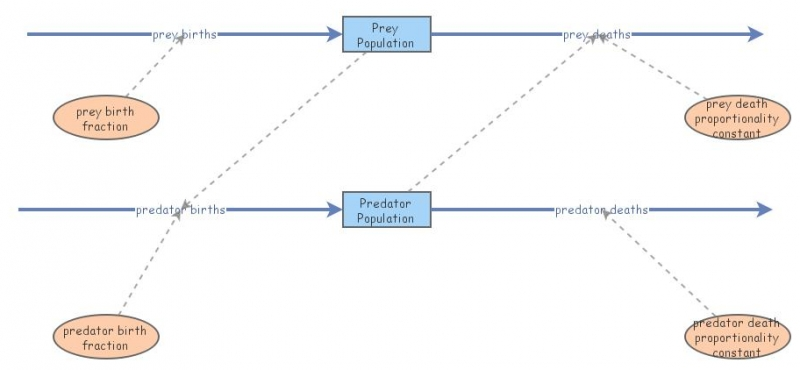Prey&PredatorPhysical meaning of the equations
The Lotka–Volterra model makes a number of assumptions about the environment and evolution of the predator and prey populations:

1. The prey population finds ample food at all times.
2. The food supply of the predator population depends entirely on the size of the prey population.
3. The rate of change of population is proportional to its size.
4. During the process, the environment does not change in favour of one species and genetic adaptation is inconsequential.
5. Predators have limitless appetite.
As differential equations are used, the solution is deterministic and continuous. This, in turn, implies that the generations of both the predator and prey are continually overlapping.

Prey
When multiplied out, the prey equation becomes
dx/dtαx - βxy
The prey are assumed to have an unlimited food supply, and to reproduce exponentially unless subject to predation; this exponential growth is represented in the equation above by the term αx. The rate of predation upon the prey is assumed to be proportional to the rate at which the predators and the prey meet; this is represented above by βxy. If either x or y is zero then there can be no predation.

With these two terms the equation above can be interpreted as: the change in the prey's numbers is given by its own growth minus the rate at which it is preyed upon.

### Predators

The predator equation becomes

dy/dt =-In this equation,represents the growth of the predator population. (Note the similarity to the predation rate; however, a different constant is used as the rate at which the predator population grows is not necessarily equal to the rate at which it consumes the prey).represents the loss rate of the predators due to either natural death or emigration; it leads to an exponential decay in the absence of prey.

Hence the equation expresses the change in the predator population as growth fueled by the food supply, minus natural death.